This book contains both practical guides on exploring missing data, as well as some of the deeper details of how `naniar` works to help you better explore your missing data. A large component of this book are the exercises that accompany each section in each chapter.

``````library(naniar)
library(tidyverse)``````
``── Attaching packages ─────────────────────────────────────── tidyverse 1.3.1 ──``
``````✔ ggplot2 3.3.6     ✔ purrr   0.3.4
✔ tibble  3.1.7     ✔ dplyr   1.0.9
✔ tidyr   1.2.0     ✔ stringr 1.4.0
✔ readr   2.1.2     ✔ forcats 0.5.1``````
``````── Conflicts ────────────────────────────────────────── tidyverse_conflicts() ──

In this chapter we discuss whether imputation is appropriate, and the features of good and bad imputations. You will learn how to evaluate imputed values by using visualisations to assess their summary features: the mean/median, scale, and spread.

## 13.1 What makes a good imputation

Imputing missing values needs to be done with care - you want to avoid imputing unlikely values like mid winter temperatures into the middle of summer, giving pigs a wing span measurement, or heavy rainfall into a known drought.

## 13.2 When to impute

Not everything can be solved with imputation. If you don’t already have the information, sometimes it is not appropriate to impute variables like, age, race, sex and gender without first confirming known facts about your population. Sometimes this means talking to the person who collected or curated the data to understand the population studied. You might learn some variables remain fixed over time, and so can be perfectly imputed. Other times, we might not know, so leaving the values as missing might be the most appropriate action. Imputation isn’t always the answer.

Another consideration for imputation is when there is simply too much missing data. For example, if you have a variable with 50% of the values missing, imputation might not be appropriate unless you have a very strong modelling case. For example, you know all the ages were not recorded for every person, but were the same for each person, so you can impute perfectly. When there are missing values in the outcome variable (the “Y”, the Dependent Variable, DV, it has many names!), it is generally not a good idea to impute data for these values. The reason is that you are effectively using the data twice.

[TODO: add a small simulation on this]

[TODO: add caveats around Bayesian/likelihood simulation approaches]

## 13.3 Understanding the good by understanding the bad

To understand good imputation, it is useful to understand bad imputations. One particularly bad imputation is mean imputation, which takes the mean of complete values as the imputed value.

For example, in a dataframe with 5 values and one missing, we calculate the mean from complete observations using `na.rm = TRUE`, and use this to impute the missing values. The steps are shown here:

``````df <- tibble(x = c(1, 4, 9, 16, NA, 36))
df``````
``````# A tibble: 6 × 1
x
<dbl>
1     1
2     4
3     9
4    16
5    NA
6    36``````
``mean(df\$x, na.rm = TRUE)``
`` 13.2``
``````df[is.na(df)] <- mean(df\$x, na.rm = TRUE)
df``````
``````# A tibble: 6 × 1
x
<dbl>
1   1
2   4
3   9
4  16
5  13.2
6  36  ``````

### 13.3.1 Demonstrating mean imputation

This is generally a terribly idea. For example, imagine we had data like this, with missing values:

``````ggplot(df,
aes(x = id,
y = x)) +
geom_point()``````
``Warning: Removed 50 rows containing missing values (geom_point).``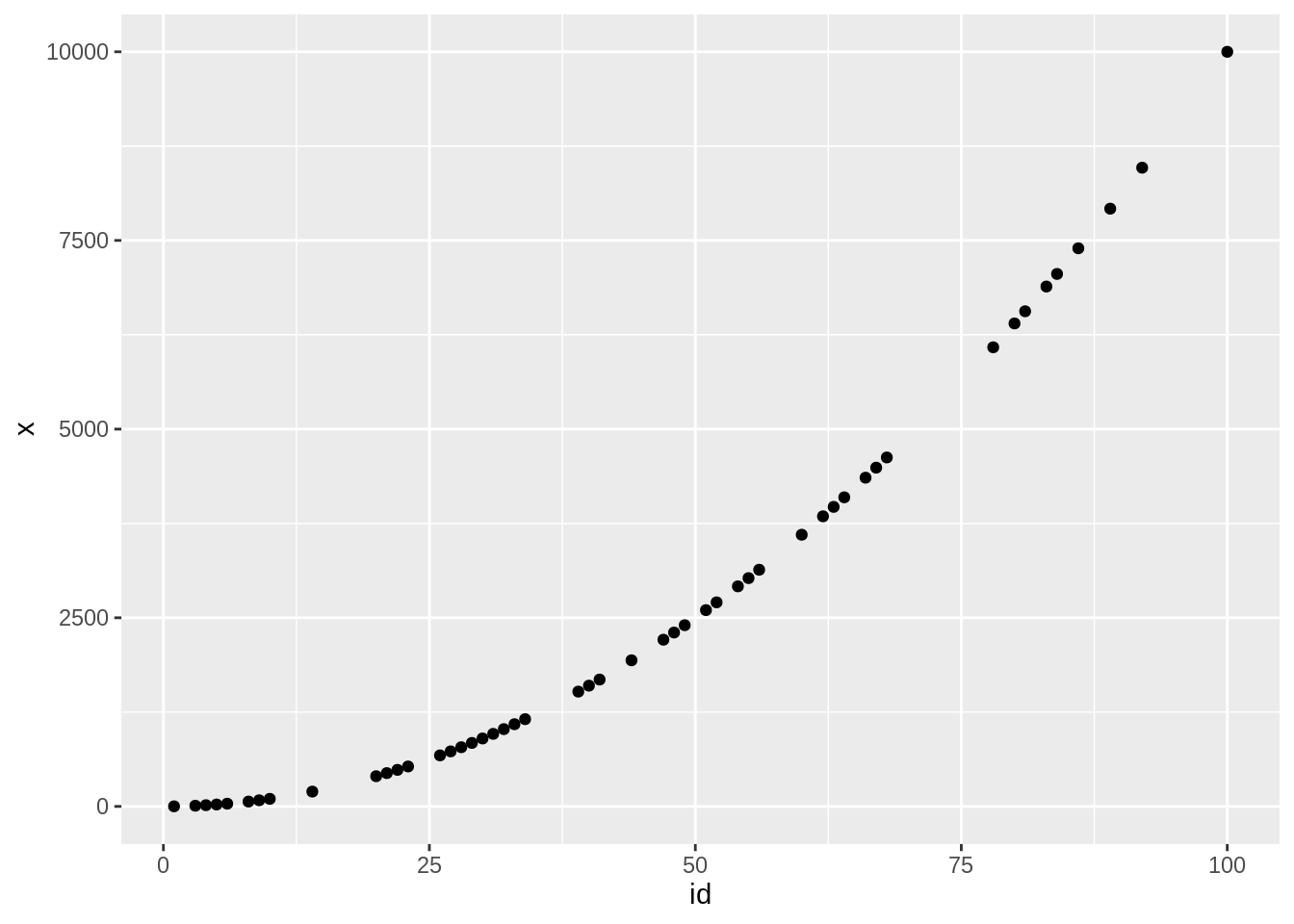Imputing the mean value in this graph, we get this:

``````df %>%
nabular() %>%
mutate(across(everything(), impute_mean)) %>%
ggplot(aes(x = id,
y = x,
colour = x_NA)) +
geom_point()``````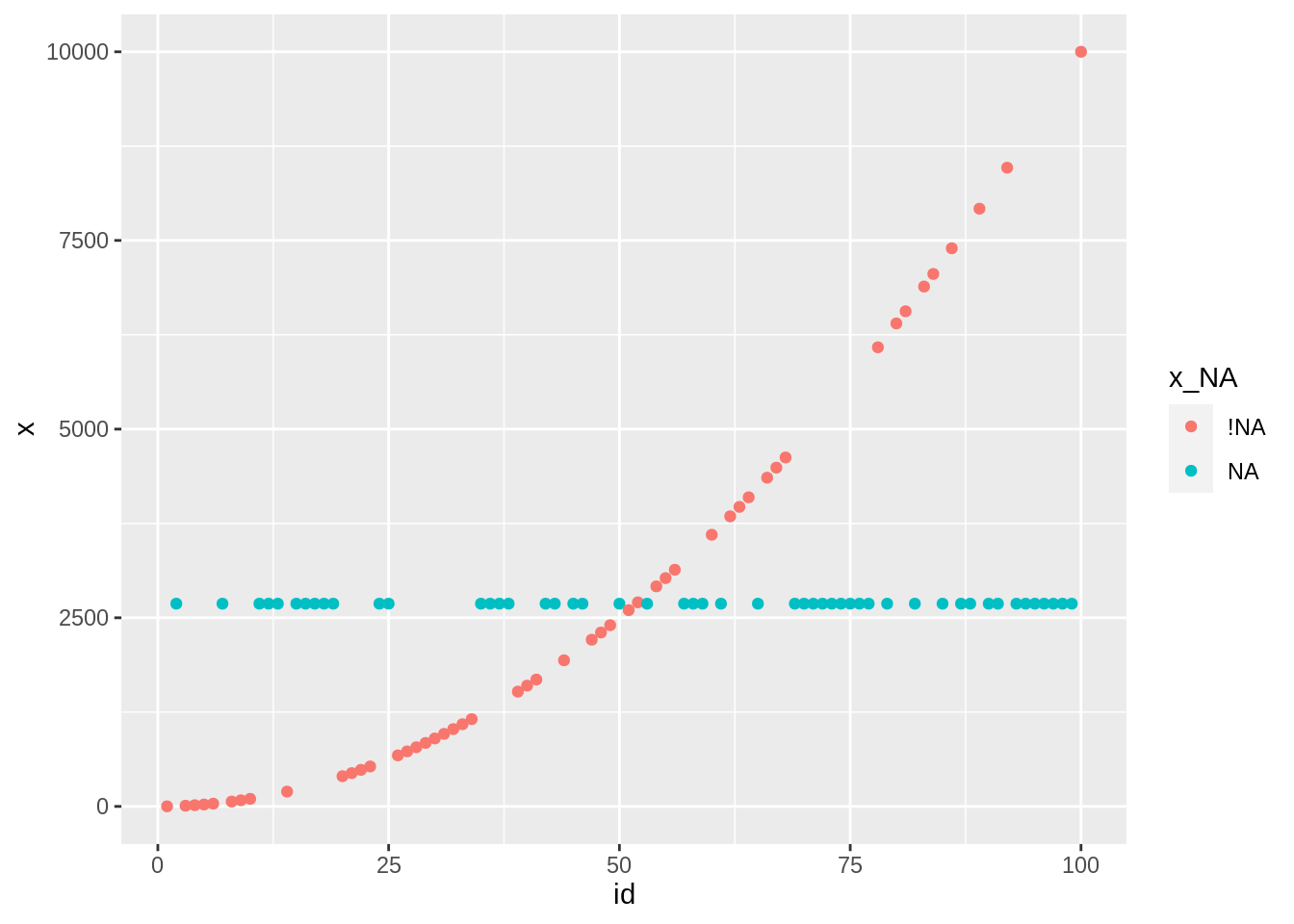The mean does not respect the underlying process of the data. Visualisation is a very key tool here to explore and demonstrate this pattern.

### 13.3.2 Explore bad imputations: The mean

To examine these bad imputations, we use the `impute_mean` function from the `naniar` package. Similar to `impute_below` used in the previous chapter, we can use `across` and friends with `impute_mean`. So it can work on a vector, on variables based on some condition like are they numeric, for specified variables, or for all variables.

### 13.3.3 Tracking missing values

To visualise imputations we use the same process as for `impute_below`:

``````aq_impute_mean <- airquality %>%
nabular(only_miss = TRUE) %>%
mutate(across(everything(), impute_mean)) %>%

aq_impute_mean``````
``````# A tibble: 153 × 9
Ozone Solar.R  Wind  Temp Month   Day Ozone_NA Solar.R_NA any_missing
<dbl>   <dbl> <dbl> <dbl> <dbl> <dbl> <fct>    <fct>      <chr>
1  41      190    7.4    67     5     1 !NA      !NA        Not Missing
2  36      118    8      72     5     2 !NA      !NA        Not Missing
3  12      149   12.6    74     5     3 !NA      !NA        Not Missing
4  18      313   11.5    62     5     4 !NA      !NA        Not Missing
5  42.1    186.  14.3    56     5     5 NA       NA         Missing
6  28      186.  14.9    66     5     6 !NA      NA         Missing
7  23      299    8.6    65     5     7 !NA      !NA        Not Missing
8  19       99   13.8    59     5     8 !NA      !NA        Not Missing
9   8       19   20.1    61     5     9 !NA      !NA        Not Missing
10  42.1    194    8.6    69     5    10 NA       !NA        Missing
# … with 143 more rows``````

We first create nabular data to track missing values. Then, we do our imputations. Then, we add a label to identify cases with missing observations using `add_label_shadow()`. One thing to have up your sleeve it `only_miss` option, which binds only columns with missing values. This makes the data bit smaller and easier to handle.

Now that we know a way to impute our data, let’s explore it. We can explore the imputed values in the same way we did for the previous lesson. But this time our intention is different, and we want to consider evaluating imputations by looking for changes in the mean, the spread, and the scale.

[TODO **A small figure/plot that clearly shows what we mean by mean, spread, scale]**

### 13.3.4 Using a boxplot to explore how the mean changes

We can evaluate changes in the mean or median using a boxplot. We put the missingness of ozone, `ozone_NA`, on the x axis, and the values of ozone on the y axis, and use `geom_boxplot`.

``````ggplot(aq_impute_mean,
aes(x = Ozone_NA,
y = Ozone)) +
geom_boxplot()``````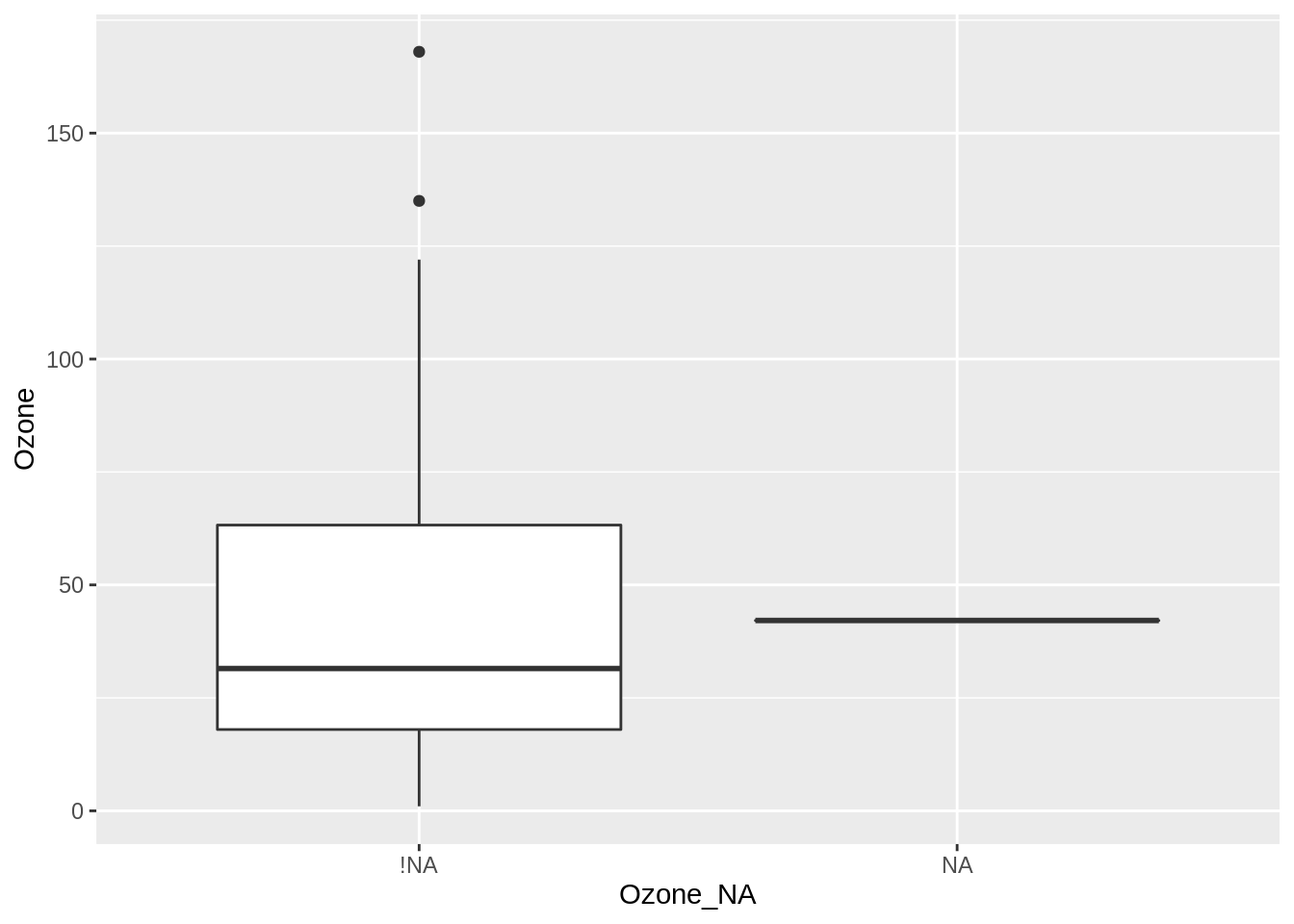From this visualisation, we learn the median value is similar in each group, but the median is lower for the not missing group. The take away message is the mean isn’t changing. This is good, but there is more than one feature to explore!

## 13.4 Using a scatterplot to Explore how spread changes with imputation

The spread of imputations can be explored using a scatter plot. We plot our airquality imputed with the mean, with Ozone and solar radiation on the x and y axis, and colouring according to missingness, `any_missing`.

``````ggplot(aq_impute_mean,
aes(x = Ozone,
y = Solar.R,
colour = any_missing)) +
geom_point()``````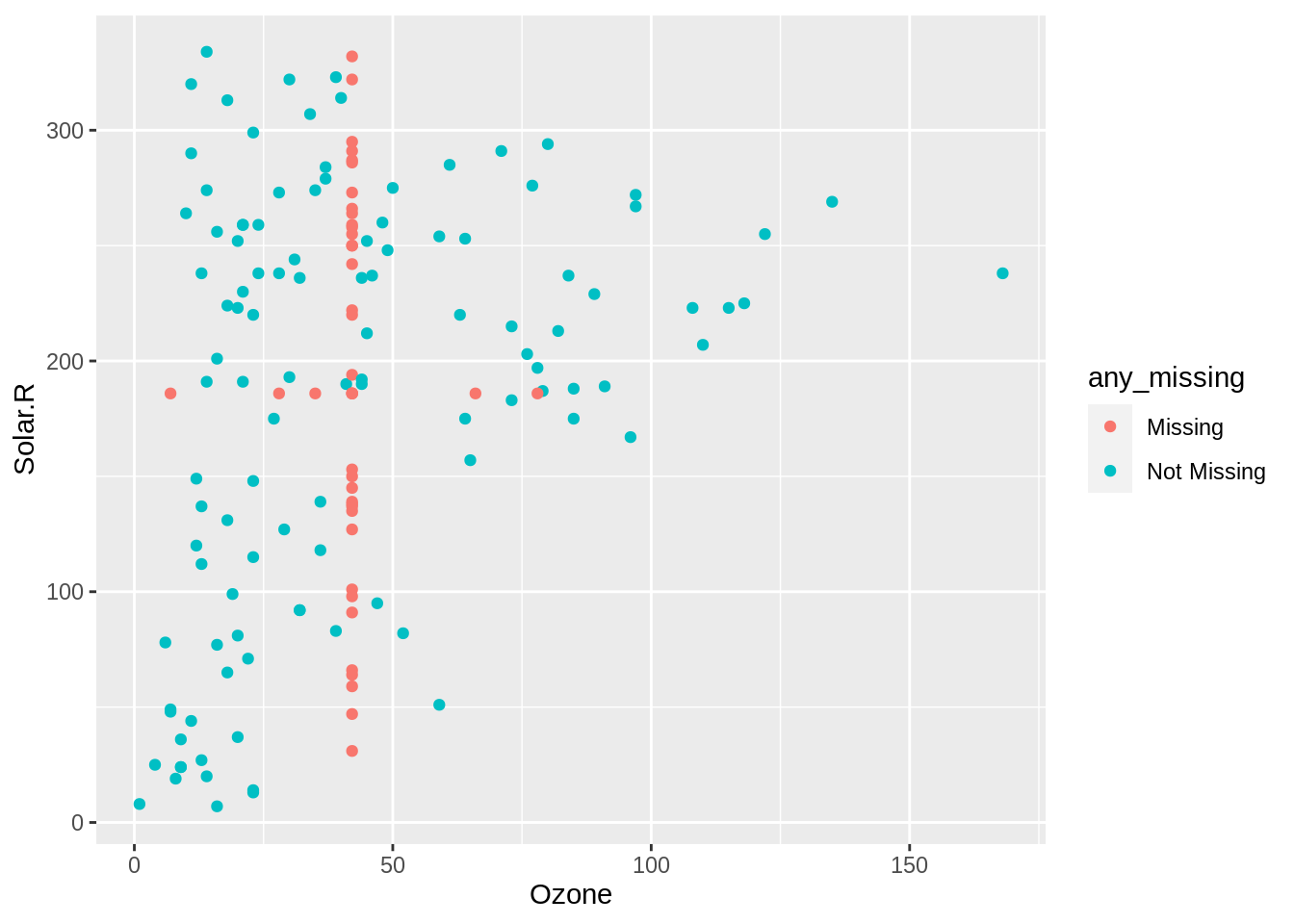We learn there is no variation in the spread of the points! Although we do notice the imputed values are within a reasonable range of the data.

### 13.4.1 How to explore imputations for many variables

``````aq_imp <- airquality %>%
nabular() %>%
mutate(across(everything(), impute_mean))``````

To make it easier to explore many variables, we use the `shadow_long` function to return `nabular` data in long format. This is similar to `pivot_longer`, but with our nabular data.

``````aq_imp_long <- shadow_long(aq_imp,
Ozone,
Solar.R)

aq_imp_long``````
``````# A tibble: 306 × 4
variable value variable_NA value_NA
<chr>    <dbl> <chr>       <chr>
1 Ozone     41   Ozone_NA    !NA
2 Ozone     36   Ozone_NA    !NA
3 Ozone     12   Ozone_NA    !NA
4 Ozone     18   Ozone_NA    !NA
5 Ozone     42.1 Ozone_NA    NA
6 Ozone     28   Ozone_NA    !NA
7 Ozone     23   Ozone_NA    !NA
8 Ozone     19   Ozone_NA    !NA
9 Ozone      8   Ozone_NA    !NA
10 Ozone     42.1 Ozone_NA    NA
# … with 296 more rows``````

Here, we enter in our data, followed by the variables that we want to focus on - in this case, `Ozone` and `Solar.R`. This returns to us data with the columns `variable`, `value`, and the shadow columns, `variable_NA` and `value_NA`.

### 13.4.2 Exploring imputations for many variables

We can then use this in a ggplot, placing `value` in the x axis, and filling by the missingness of the value, `value_NA`, and then using `geom_histogram`, facetting by `variable`.

``````ggplot(aq_imp_long,
aes(x = value,
fill = value_NA)) +
geom_histogram() +
facet_wrap(~variable)``````
```stat_bin()` using `bins = 30`. Pick better value with `binwidth`.``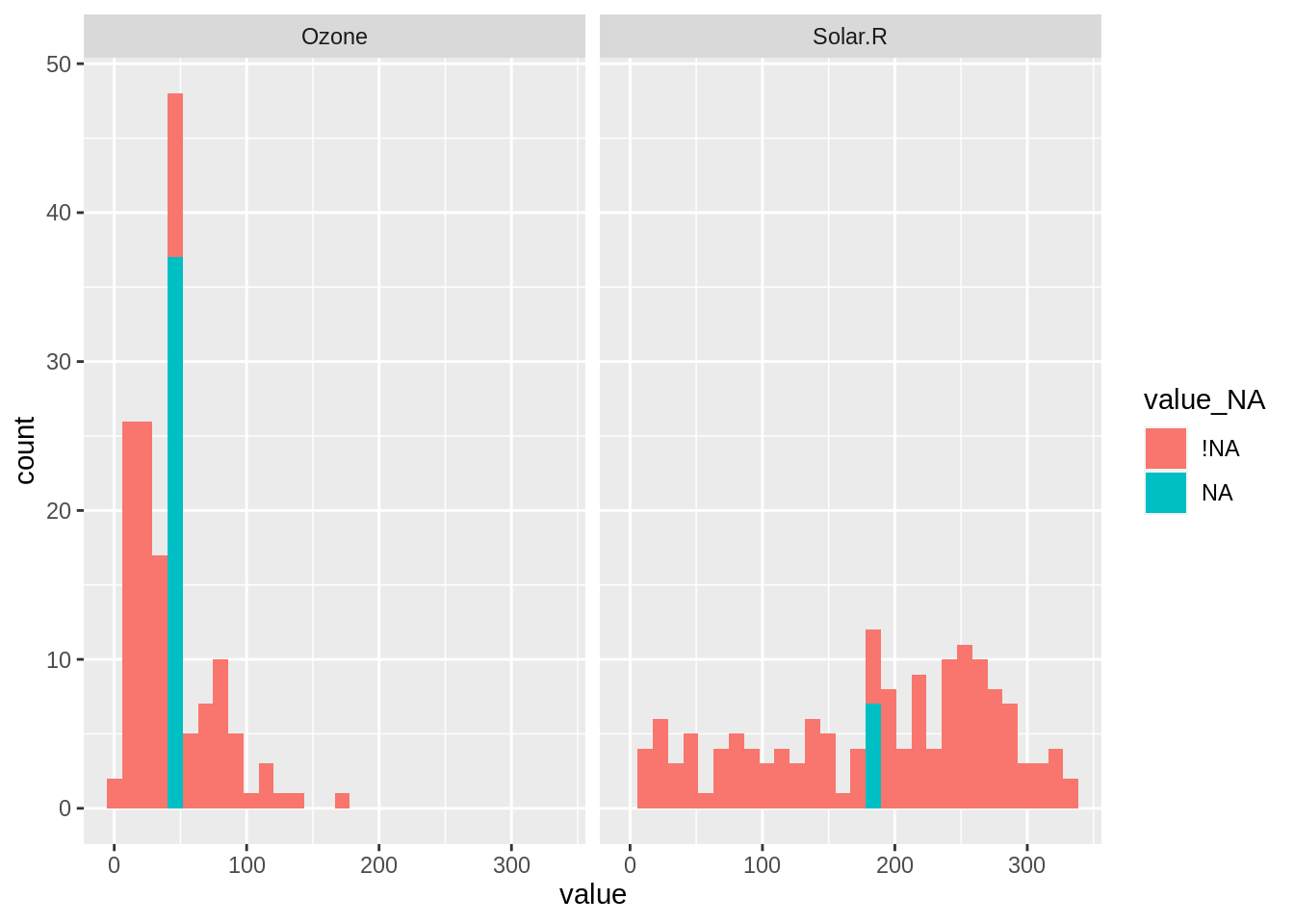The format from `shadow_long` makes it simpler to explore missing values for many variables.

[TODO perhaps another example of this with other data]